Ministério da Ciência, Tecnologia, Inovações e Comunicações CBPFIndex CENTRO BRASILEIRO DE PESQUISAS FÍSICAS11/12/2023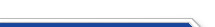Usuário: Senha: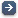Crie sua conta aqui · Busca rápida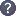Procurar por: Publicações Projetos EventosIr para a busca avançada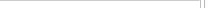Notas de Física
 New aspects of the ${\mathbb Z}_2\times {\mathbb Z}_2$-graded $1D$ superspace: induced strings and $2D$ relativistic models Data do cadastro: 13/02/2023 Publicação/Divulgação: 13/02/2023 Resp. pelo cadastro: Status atual: Publicado Ano da publicação: 2022 Descrição: A novel feature of the ${\mathbb Z}_2\times {\mathbb Z}_2$-graded supersymmetry which finds no counterpart in ordinary supersymmetry is the presence of $11$-graded exotic bosons (implied by the existence of two classes of parafermions). Their interpretation, both physical and mathematical, presents a challenge. The role of the exotic bosonic coordinate" was not considered by previous works on the one-dimensional \zzg superspace (which was restricted to produce point-particle models). By treating this coordinate at par with the other graded superspace coordinates new consequences are obtained. The graded superspace calculus of the \zzg worldline super-Poincar\'e algebra induces two-dimensional \zzg relativistic models; they are invariant under a new \zzg $2D$ super-Poincar\'e algebra which differs from the previous two \zzg $2D$ versions of super-Poincar\'e introduced in the literature. In this new superalgebra the second translation generator and the Lorentz boost are $11$-graded. Furthermore, if the exotic coordinate is compactified on a circle ${\bf S}^1$, a \zzg closed string with periodic boundary conditions is derived. \\ The analysis of the irreducibility conditions of the $2D$ supermultiplet implies that a larger $(\beta$-deformed, where $\beta\geq 0$ is a real parameter) class of point-particle models than the ones discussed so far in the literature (recovered at $\beta=0$) is obtained. While the spectrum of the $\beta=0$ point-particle models is degenerate (due to its relation with an ${\cal N}=2$ supersymmetry), this is no longer the case for the $\beta> 0$ models. Número: CBPF-NF-004/22 Autores: N. Aizawa; R. Ito; Z. Kuznetsova; F. Toppan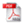Download do PDF (1.192 KB)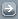Todos EventosTodos PublicaçõesTodasArtigos de Div. CientíficaArtigos em Revistas Nac.Artigos em Revistas Int.Artigos em Eventos Nac.Artigos em Eventos Int.Livros InteirosCapítulos de LivroPropriedades IntelectuaisÁudioPatentesProgramas de computadorModelos de utilidadeMarcasOutras prop. intel.TecnologiasKnow-howServiços tecnológicosTecnologias inovadorasTeses de DoutoradoDissertações de MestradoProjetos de GraduaçãoCiência e SociedadeDocumentos HistóricosMonografiasNotas de FísicaNotas TécnicasOutras Produções
 · Principal    |    CBPF    |    · Copyright 2004 - CBPF. Rio de Janeiro/BrasilCentro Brasileiro de Pesquisas Físicasv 1.1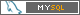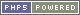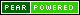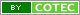Pï¿½g. gerada em 0.01 s Total de consultas SQL: 31